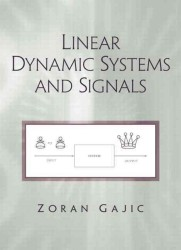Linear Dynamic Systems and Signals

• ただいまウェブストアではご注文を受け付けておりません。 ⇒古書を探す
• 製本 Hardcover:ハードカバー版／ページ数 700 p.
• 言語 ENG
• 商品コード 9780201618549
• DDC分類 621.3822

基本説明

ＭＡＴＬＡＢによる演習事例を収録。

Full Description

For sophomore- and junior-level courses in Linear Systems and Signals for electrical engineering, biomedical engineering or mechanical engineering majors.

The author's experience teaching undergraduate- and graduate-level linear systems courses for more than 15 years is reflected in this comprehensive text. It contains detailed linear system theory essentials and presents and develops the unified techniques to recognize and solve linear dynamical system problems regardless of their origin. With this text, students will be well equipped to cope with all types of linear dynamic system problems that may be encountered.

Contents

Preface.

1. Introduction to Linear Systems.

1.1 Continuous and Discrete Linear Systems and Signals. 1.2 System Linearity and Time Invariance. 1.3 Mathematical Modeling of Systems. 1.4 System Classification. 1.5 MATLAB System Computer Analysis and Design. 1.6 Book Organization. 1.7 Chapter One Summary. 1.8 References. 1.9 Problems.

2. Introduction to Signals.

2.1 Common Signals in Linear Systems. 2.2 Signal Operations. 2.3 Signal Classification. 2.4 MATLAB Laboratory Experiment on Signals. 2.5 Chapter Two Summary. 2.6 References. 2.7 Problems.

I. FREQUENCY DOMAIN TECHNIQUES.

3. Fourier Series and Fourier Transform.

3.1 Fourier Series. 3.2 Fourier Transform and Its Properties. 3.3 Fourier Transform in System Analysis. 3.4 Fourier Series in Systems Analysis. 3.5 From Fourier Transform to Laplace Transform. 3.6 Fourier Analysis MATLAB Laboratory Experiment. 3.7 Chapter Three Summary. 3.8 References. 3.9 Problems.

4. Laplace Transform.

4.1 Laplace Transform and Its Properties. 4.2 Inverse Laplace Transform. 4.3 Laplace Transform in Linear System Analysis. 4.4 Block Diagrams. 4.5 From Laplace to the z-Transform. 4.6 MATLAB Laboratory Experiment. 4.7 Chapter Four Summary. 4.8 References. 4.9 Problems.

5. The z Transform.

5.1 The z Transform and Its Properties. 5.2 Inverse of the z Transform. 5.3 The z Transform in Linear System Analysis. 5.4 Block Diagram. 5.5 Discrete-Time Frequency Spectra. 5.6 MATLAB Laboratory Experiment. 5.7 Chapter Five Summary. 5.8 References. 5.9 Problems.

II. TIME DOMAIN TECHNIQUES.

6. Convolution.

6.1 Convolution of Continuous-Time Signals. 6.2 Convolution for Linear Continuous-Time Systems. 6.3 Convolution of Discrete-Time Signals. 6.4 Convolution for Linear Discrete-Time Systems. 6.5 Numerical Convolution Using MATLAB. 6.6 MATLAB Laboratory Experiments on Convolution. 6.7 Chapter Six Summary. 6.8 References. 6.9 Problems.

7. System Response in Time Domain.

7.1 Solving Linear Differential Equations. 7.2 Solving Linear Difference Equations. 7.3 Discrete-Time System Impulse Response. 7.4 Continuous-Time System Impulse Response. 7.5 Complete Continuous-Time System Response. 7.6 Complete Discrete-Time System Response. 7.7 Stability of Continuous-Time Linear Systems. 7.8 Stability of Discrete-Time Linear Systems. 7.9 MATLAB Experiment on Continuous-Time Systems. 7.10 MATLAB Experiment on Discrete-Time Systems. 7.11 Chapter Seven Summary. 7.12 References. 7.13 Problems.

8. State Space Approach.

8.1 State Space Models. 8.2 Time Response from the State Equation. 8.3 Discrete-Time Models. 8.4 System Characteristic Equation and Eigenvalues. 8.5 Cayley-Hamilton Theorem. 8.6 Linearization of Nonlinear System. 8.7 State Space MATLAB Laboratory Experiments. 8.8 Chapter Eight Summary. 8.9 References. 8.10 Problems.

III. SYSTEMS IN ELECTRICAL ENGINEERING.

9. Signals in Digital Signal Processing.

9.1 Sampling Theorem. 9.2 Discrete-Time Fourier Transform (DFDT). 9.3 Double Sided z-Transform. 9.4 Discrete Fourier Transform. 9.5 Discrete-Time Fourier Series. 9.6 Correlation of Discrete-Time Signals. 9.7 FIR and IIR Filters. 9.8 Laboratory Experiment on Digital Signal Processing. 9.9 Chapter Nine Summary. 9.10 References. 9.11 Problems.

10. Signals in Communication Systems.

10.1 Signal Transmission in Communications. 10.2 Signal Correlation, Energy and Power Spectra. 10.3 Hilbert Transform. 10.4 Ideal Filter. 10.5 Modulation and Demodulation. 10.6 Digital Communication System. 10.7 Communication Systems Laboratory Experiment. 10.8 Chapter Ten Summary. 10.9 References. 10.10 Problems.

11. Linear Electric Circuits.

11.1 Basic Relations. 11.2 First-Order Linear Electrical Circuits. 11.3 Second-Order Linear Electrical Circuits. 11.4 Higher-Order Linear Electrical Circuits. 11.5 Chapter Eleven Summary. 11.6 References. 11.7 MATLAB Laboratory Experiment. 11.8 Problems.

12. Linear Controls Systems.

12.1 The Essence of Feedback. 12.2 Transient Response of Second-Order Systems. 12.3 Feedback System Steady State Errors. 12.4 Feedback System Frequency Characteristics. 12.5 Bode Diagrams. 12.6 Common Dynamic Controllers: PD, PI, PID. 12.7 Laboratory Experiment on Control Systems. 12.8 Chapter Twelve Summary. 12.9 References. 12.10 Problems.

Appendices.

A. Linear Algebra. B. Some Results from Calculus. C. Introduction to MATLAB. D. Introduction to SIMULINK.

Index.